Disclaimer: This is an example of a student written essay.

Any scientific information contained within this essay should not be treated as fact, this content is to be used for educational purposes only and may contain factual inaccuracies or be out of date.

# Stoichiometry Calculations Essay

 ✅ Paper Type: Free Essay ✅ Subject: Chemistry ✅ Wordcount: 1477 words ✅ Published: 30th Nov 2017

• Regina Marin

Chapter 12:

Stoichiometry

SECTION 1 – WHAT IS STOICHIOMETRY?

Mole-Mass Relationships in chemical reactions

The balance equations is the main basis of stoichiometry, and that is the first thing to do when you want to measure a chemical process. The information provided by the chemical equation is interpreted according to the needs. And the mole is associated with the molecular weight, the molar ratios of an equation can be associated with certain of the substances involved by the mol mass. The transformations that occur in a chemical reaction is governed by the law of conservation of mass: Atoms are neither created nor destroyed during a chemical reaction.

Let`s try to interpret an equation in terms of moles, particles, and mass.

Remeber 2 important facts: – The coefficient indicates the amount of molecules

The coefficient also indicates the amount of moles

Then lets get the mass of the reactants and the products by using conversion.

Moles reactant/product * grams reactant/product / 1 mole reactant/product = grams reactant

Mole Ratios

We know that there is a direct relationship between mole and molecule. This relationship is Avogadro’s number. A mole always contains Avogadro’s number of molecules. Thus the relationships between molecules given by the stoichiometric coefficients are also valid for moles. When a chemical reaction in the molar ratios of reactants and products are given, if you can have fractional stoichiometric coefficients, since the amounts of molecules involved are very high, and we can talk about half mole of molecules or of a third mole of molecules.

Stoichiometric coefficients indicate the proportion that the moles of reactants and products involved in a chemical reaction.

SECTION 2 – STOICHIOMETRIC CALCULATIONS

Stoichiometric mole-to-mole Conversion

In this type of reaction the starting material is expressed in moles, and the desired substance are requested in moles. In stoichiometric calculations results are reported be rounded to two decimals. Similarly, the atomic masses of elements should be used rounded to two decimals.

Recalling: Rounding to two decimal places, we use as a basis the third decimal. If this is greater than or equal to 5, increase one unit to two decimal places; if less than or equal to 4 the second decimal lacifra be retained.

So let`s see the steps to make a mole to mole conversion:

STEP 1: Balancing the equation

Reviewing the equation we make sure that is really well balanced. We can represent the balanced equation in the data and the unknown exercise.

STEP 2: Identify

We need to identify the substance that we are looking for and the one that we are starting of.

STEP 3: Apply the mole ratio.

The moles of desired substance and starting the get from the balanced equation.

Stoichiometric mole-to-mas Conversion

To find the mass of a product, is enough to multiply the moles of the product by it`s molecular weight in g / mol.

Stoichiometric mass-to-mass conversion

Molar factor method is based on the ratio of the number of moles between two substances participating in a chemical reaction.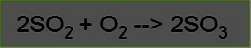Step 1: Balance the equation

Step 2: Determine the molar factor, which is equal to the number of moles searched on the number of moles known.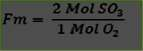Step 3: Find the moles of O2 from O2 500g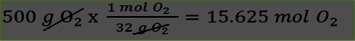Step 4: Find the moles of SO3 from the O2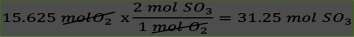Step 5: We find the grams of SO3 after the SO3 mol 31.25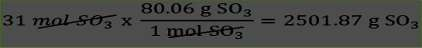SECTION 3 – LIMITING REACTANTS

Why do Reactions Stop?

When adjusted equation, the coefficients represent the number of atoms of each element in the reactants and the products. Also represent the number of molecules and moles of reactants and products. When an equation is set, the stoichiometry is used to find the moles of a product obtained from a known number of moles of a reagent. The mole ratio between reactant and product is obtained from the fitted equation. Sometimes mistakenly believes that the reactions are always used the exact amounts of reagents. However, in normal practice it is usually an excess of one or more reactants are used, to ensure that the widest possible to react the less abundant reactive.

Limiting Reactants

When a reaction is stopped because just one of the reactants, that reactant is called the limiting reactant. One reactant that has been completely consumed in a chemical reaction is known by the name of limiting reagent as it determines or limits the amount of product formed.

“Limiting reagent is one that is in default based on the balanced chemical equation”

2H2 + O2 → 2H2O

In the equation above, What is the limiting reactant if we have 10 molecules of hydrogen and 10 oxygen molecules?

Need 2 molecules per molecule of H2 O2

But we only have 10 molecules of H2 and 10 molecules of O2.

The required ratio is 2: 1

But we have the proportion is 1: 1

It is clear that the excess reagent is the limiting reactant O2 and H2 is

How to work with molecules is the same as working with moles.

If we now put 15 moles of H2 with 5 moles of O2 so as the stoichiometry of the reaction is such that 1 mol of O2 reacts with 2 moles of H2, then the number of moles of O2 needed to react with all the H2 is 7, 5, and the number of moles of H2 required to react with all the O2 is 10.

That is, that after all the oxygen has been consumed, 5 moles of hydrogen left over. The O2 is the limiting reactant

One way to solve the problem of what is the limiting reagent is:

Calculate the amount of product to be formed for each of the quantities to the reaction reagent.

The limiting reactant is one that produces the least amount of product.

Excess Reactant

The reactant is completely consumed is called the limiting reagent because it determines the amount of product that can be produced in the reaction. When the limiting reactant is consumed, the reaction is stopped.

The reactant reacts not completely, but “plenty”, is called the excess reagent. If we have a certain amount two elements or compounds to produce a chemical reaction, we can know in advance what will be the limiting reagent and excess reagent which, making some calculations based on the balanced chemical equation.

SECTION 4 – PERCENT YIELD

How much product?

When the stoichiometric calculations for finding as a substance produced in a chemical reaction is made, it is expected that the amount produced of the substance will always be less than the amount of the substance to be obtained , this happens because not everything reacts completely converted to product , ie not all that does react 100% . Because of this, in stoichiometry concept yield percentage is included , the percent yield is a chemical reaction is the ratio between the actual performance and theoretical performance, the ratio of grams that actually occur in the reaction and grams that would be obtained if all you react is transformed into product.

Our academic experts are ready and waiting to assist with any writing project you may have. From simple essay plans, through to full dissertations, you can guarantee we have a service perfectly matched to your needs.

If a problem involving a chemical reaction term refers to the theoretical yield of a reaction means that we take as if everything becomes reactive product , otherwise we would be talking about an actual performance. The equation used to solve problems that involve the performance of a chemical reaction is as follows: . % Yield = (actual yield ( g ) ) / (theoretical yield ( g ) ) × 100 % If a problem of chemical reaction we give grams that actually produce a substance by reacting certain amount of reactant either molar or mass , it can find the percentage yield of the reaction using this equation, because they would not be given the actual performance and theoretical performance assuming that the substance would find completely react .

View all

## DMCA / Removal Request

If you are the original writer of this essay and no longer wish to have your work published on UKEssays.com then please:

Related Services

Related Lectures